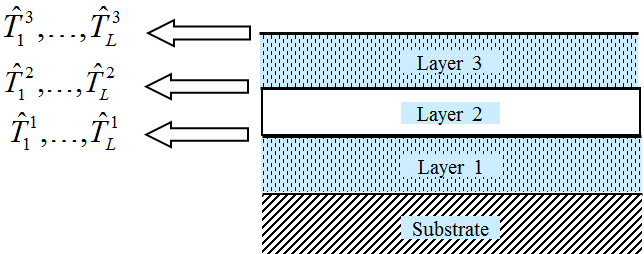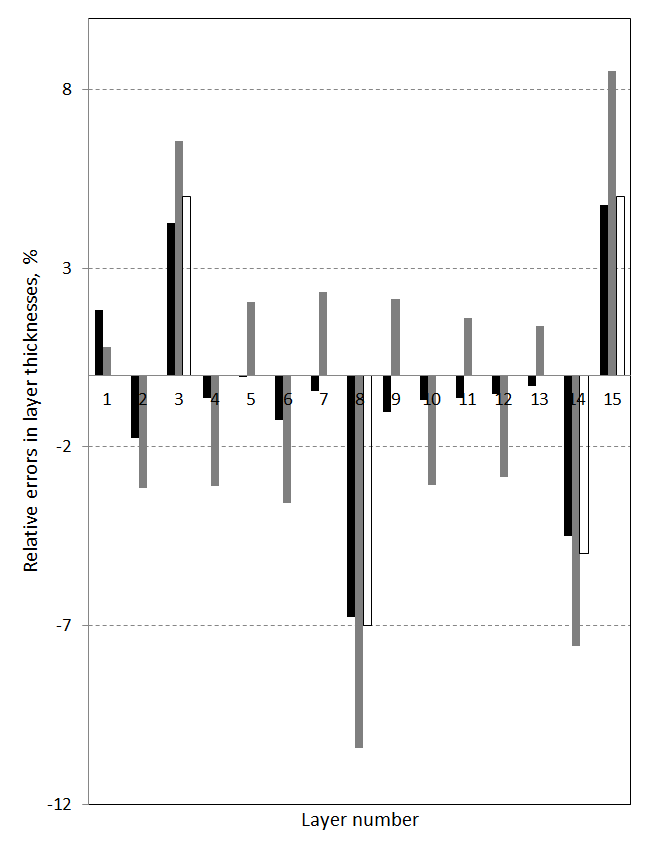### On-line characterization

 The main goal of the on-line characterization is the determination of thicknesses of already deposited layers. On-line characterization functions of OptiReOpt DLL can be also used for monitoring deposition processes by generating a command for the termination of current layer deposition. The on-line characterization of thicknesses of already deposited layers requires in situ measurements of spectral transmittance and/or spectral reflectance just after the end of deposition of each coating layer. If OptiReOpt DLL is used for the monitoring purposes, then in situ spectral scans of coating transmittance and/or reflectance should be done during layer depositions in small time intervals that can provide a sufficient accuracy of layer thickness monitoring.$$\hat{T}^j_1,...,\hat{T}^j_L$$ in situ spectral monitoring data collected after the deposition of j-th layer ($$L$$ – total number of data points in one spectral scan) There are two computational modes of the OptiReOpt characterization routines. These modes are referred to as SEQUENTIAL and TRIANGLE. In the case of SEQUENTIAL mode only thickness of the last deposited layer is determined by the characterization routine.   It is advisable to use SEQUENTIAL mode only in the case of very stable deposition processes and high-accuracy in situ measurement data. Discrepancy function (SEQUENTIAL algorithm): $DF(d_i)=\left(\frac 1L \sum_{j=1}^L\left[ \frac{\hat{T}(\hat{d}_1,...,\hat{d}_{i-1}, d_i;\lambda_j)-\hat{T}^{(i)}(\lambda_j)}{\Delta T_j}\right]^2\right)^{1/2},$ where $$\hat{d}_1,...,\hat{d}_{i-1}$$ are the thicknesses of the previously deposited layers that were determined at the previous steps of the algorithm. The discrepancy functions are minimized with respect to only one variable: $DF(i)\rightarrow \min$ Because of this fact, this algorithm usually works faster than the triangular algorithm. This is of course an attractive feature of the sequential algorithm. At the same time,  this algorithm may suffer fromthe effect of accumulation of thickness errors. In the case of TRIANGLE mode thicknesses of all already deposited layers are determined at each characterization step. Operational time of OptiReOpt DLL in SEQUENTIAL mode is faster than in TRIANGLE mode. At the same time, in general, TRIANGLE mode provides more reliable thicknesses of all already deposited layers. Discrepancy function (TRIANGULAR algorithm): $DF(i)=\left(\frac 1i \sum_{k=1}^i \frac 1L\sum_{j=1}^L\left[ \frac{\hat{T}(d_1,..., d_i;\lambda_j)-\hat{T}^{(k)}(\lambda_j)}{\Delta T_j}\right]^2\right)^{1/2},$ The discrepancy functions are minimized with respect to thicknesses of the first $$k$$ deposited layers: $DF(d_1,...,d_i)\rightarrow \min$ Example. 15-layer quarter-wave mirror was deposited with imposed errors in layer thicknesses. Thickness errors of +7%, -7%, -5% and +5% were done in layers 3, 8, 14 and 15 (empty bars on the right panel). Sequential algorithm provides errors' estimations that do not correlate with the imposed errors (grey bars). Triangular algorithm determines thickness errors with high accuracy (black bars).  There are other types of algorithms, for example, hybrid algorithms where at each step errors in several previous layers are determined. Automation tool of OptiLayer can help you to find the algorithm appropriate for your production environment. You can easily create your own program which will call OptiRE from your program with various setting/models and develop the most appropriate optical coating model. This model can be later used in on-line characterization with OptiReOpt.References: T.V. Amotchkina, M.K. Trubetskov, V. Pervak, S.Schlichting, H. Ehlers, D. Ristau, and A.V. Tikhonravov. "Comparison of algorithms used for optical characterization of multilayer optical coatings," Appl. Opt. 50, 3389-3395 (2011). A. V. Tikhonravov, M. K. Trubetskov, "Online characterization and reoptimization of optical coatings", Proc. SPIE. 5250, Advances in Optical Thin Films 406 (2004) S. Wilbrandt, O. Stenzel, N. Kaiser, M.K. Trubetskov, and A.V. Tikhonravov, "In situ optical characterization and reengineering of interference coatings," Appl. Opt. 47, C49-C54 (2008). S. Wilbrandt, O. Stenzel, N. Kaiser, M. K. Trubetskov, and A. V. Tikhonravov, "On-line Re-engineering of Interference Coatings," in Optical Interference Coatings, OSA Technical Digest (CD) (Optical Society of America, 2007), paper WC10. J. Oliver, A. Tikhonravov, M. Trubetskov, I. Kochikov, and D. Smith, "Real-Time characterization and optimization of e-beam evaporated optical coatings," in Optical Interference Coatings, OSA Technical Digest Series (Optical Society of America, 2001), paper ME8.

### Easy to startOptiLayer provides user-friendly interface and a variety of examples allowing even a beginner to effectively start to design and characterize optical coatings.        Read more...

### Docs / SupportComprehensive manual in PDF format and e-mail support help you at each step of your work with OptiLayer.If you are already an experienced user, OptiLayer gives your almost unlimited opportunities in solving all problems arising in design-production chain. Visit our publications page and challenge page.